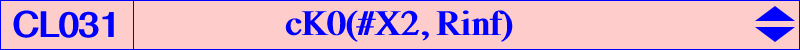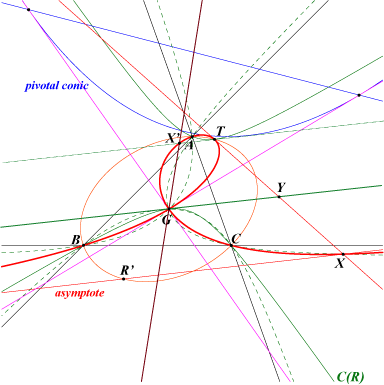For any point R on the line at infinity, the cubic cK0(#X2, R) is an isotomic nodal cubic with node G, locus of point M such that the tripolar centroid (see CL029) of M lies on the line GR. See a generalization in the page P-conical cubics. The cubic contains : T trilinear pole of the line GR, a point on the Steiner ellipse, T' isotomic conjugate of T, a point on the line at infinity, S1 and S2, (imaginary) points at infinity of the Steiner ellipse The nodal tangents are the parallels at G to the asymptotes of the circum-conic C(R) with perspector R, passing through T and G, these two points being antipodes on the conic. These tangents are perpendicular if and only if C(R) is a rectangular hyperbola i.e. if and only if R lies on the orthic axis. Hence, there is only one such cubic with rectangular nodal tangents : it is K185, the Hirst-Kiepert cubic. Note that the center of C(R) lies on the trilinear polar of R intersecting the tangent at G to C(R) at Y. This cubic always has one real asymptote intersecting the cubic again at X, reflection of T in Y, and two imaginary asymptotes meeting at T on the cubic. This real asymptote is the homothetic of the tangent at G to C(R) under h(T, 2). The tangent at T to the cubic passes through X. Naturally, the isotomic conjugate X' of X lies on the curve and is the intersection of GR and the parallel at T to the real asymptote. This configuration is very much alike that of focal cubics.The pivotal conic of cK0(#X2, R) is always a parabola whose axis is parallel to the line GR. It is inscribed in the antimedial triangle and is tangent to the nodal tangents. The points of tangency are the intersections of the pivotal conic with the polar line L of G in this conic. L contains the real inflexion point of the cubic. This parabola is the anticomplement of the parabola inscribed in ABC whose perspector is R', the isotomic conjugate of R and whose focus is the isogonal conjugate of R. R' lies on the real asymptote and is the antipode of T on the Steiner ellipse. cK0(#X2, R) can be seen as the locus of M such that the midpoint of two isotomic points M and M' lies on the tangent at G to C(R) which is the polar line of R in the Steiner ellipse. *** Let (L1), (L2) be two parallels to the polar line of R in the Steiner ellipse and symmetric in G. Let (C1), (C2) be their respective isotomic transforms. (L1), (C2) meet at M1, N1 and (L2), (C1) meet at M2, N2. These four points lie on cK0(#X2, R).cK0(#X2, R) is also the transform of C(R) in the Hirst transformation with pole G and conic the Steiner ellipse. The polar lines of two antipodes m, m' in the Steiner ellipse meet the lines Gm, Gm' at the isotomic conjugates M, M' on the cubic and these polar lines meet on the polar line of G in C(R). More over, they are tangent to the pivotal conic. Let M be a variable point on the conic C(R). The parallels at M to the cevian lines of tR (isotomic conjugate of R, a point on the Steiner ellipse) meet the sidelines of ABC at three collinear points on a line L(M). The trilinear pole of L(M) lies on the cubic cK0(#X2, R). *** The following table gives a selection of such cubics containing at least three centers.R centers on the cubic cubic X(30) X(2), X(525), X(648) X(512) X(2), X(385), X(538), X(1916) X(513) X(2), X(239), X(335), X(536) K1305 X(514) X(2), X(291), X(350), X(447), X(519), X(903) K296 X(519) X(2), X(190), X(514) X(520) X(2), X(401), X(1972) X(521) X(2), X(448), X(1944), X(1952) X(522) X(2), X(527), X(673), X(1121), X(1948) K1304 X(523) X(2), X(287), X(297), X(524), X(671), X(694) K185 X(524) X(2), X(99), X(523) X(525) X(2), X(30), X(98), X(325), X(1494) K953 X(527) X(2), X(522), X(664) X(528) X(2), X(666), X(918) X(536) X(2), X(513), X(668) X(537) X(2), X(812), X(1022) X(538) X(2), X(512), X(670) X(542) X(2), X(2394), X(2407) X(543) X(2), X(690), X(892) X(782) X(2), X(32), X(1502) X(794) X(2), X(31), X(561) X(804) X(2), X(6), X(76) X(812) X(1), X(2), X(75), X(537) X(918) X(2), X(7), X(8), X(528)